Courses

# Forces - 2 Notes | EduRev

## Civil Engineering (CE) : Forces - 2 Notes | EduRev

The document Forces - 2 Notes | EduRev is a part of Civil Engineering (CE) category.
All you need of Civil Engineering (CE) at this link: Civil Engineering (CE)

Gravitation

Gravity forces acting on masses that are a large distance apart Consider two masses m1 and m2 that are a distance d apart. Newton’s law of gravitation states that mass m1 will experience a force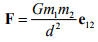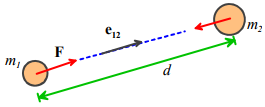where e12 is a unit vector pointing from mass m1 to mass m2 , and G is the Gravitation constant. Mass m2 will experience a force of equal magnitude, acting in the opposite direction.

In SI units, G = 6.673×10−11 m3kg -1s-2

The law is strictly only valid if the masses are very small (infinitely small, in fact) compared with d – so the formula works best for calculating the force exerted by one planet or another; or the force exerted by the earth on a satellite.

Gravity forces acting on a small object close to the earth’s surface

For engineering purposes, we can usually assume that

1. The earth is spherical, with a radius R

2. The object of interest is small compared with R

3. The object’s height h above the earths surface is small compared to R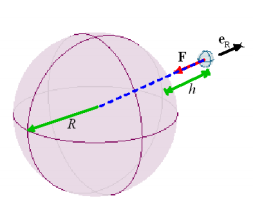If the first two assumptions are valid, then one can show that Newton’s law of gravitation implies that a mass m at a height h above the earth’s surface experiences a force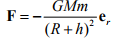where M is the mass of the earth; m is the mass of the object; R is the earth’s radius, G is the gravitation constant and er is a unit vector pointing from the center of the earth to the mass m. (Why do we have to show this? Well, the mass m actually experiences a force of attraction towards every point inside the earth. One might guess that points close to the earth’s surface under the mass would attract the mass more than those far away, so the earth would exert a larger gravitational force than a very small object with the same mass located at the earth’s center. But this turns out not to be the case, as long as the earth is perfectly uniform and spherical).

If the third assumption (h<<R) is valid, then we can simplify the force law by setting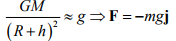where g is a constant, and j is a `vertical’ unit vector (i.e. perpendicular to the earth’s surface).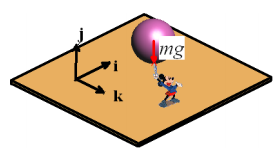In SI units g = 9.81ms−2 .

The force of gravity acts at the center of gravity of an object. For most engineering calculations the center of gravity of an object can be assumed to be the same as its center of mass. For example, gravity would exert a force at the center of the sphere that Mickey is holding. The location of the center of mass for several other common shapes is shown below. The procedure for calculating center of mass of a complex shaped object is discussed in more detail in section 6.3.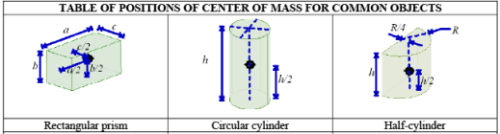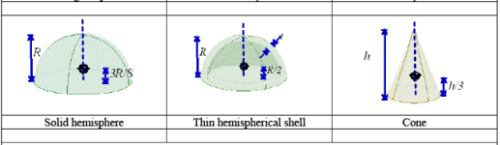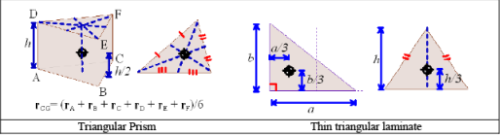There are some situations where the simple equations in the preceding section don’t work. Surveyors know perfectly well that the earth is no-where near spherical; its density is also not uniform. The earth’s gravitational field can be quite severely distorted near large mountains, for example. So using the simple gravitational formulas in surveying applications (e.g. to find the `vertical’ direction) can lead to large errors.

Also, the center of gravity of an object is not the same as its center of mass. Gravity is actually a distributed force. When two nearby objects exert a gravitational force on each other, every point in one body is attracted towards every point inside its neighbor. The distributed force can be replaced by a single, statically equivalent force, but the point where the equivalent force acts depends on the relative positions of the two objects, and is not generally a fixed point in either solid. One consequence of this behavior is that gravity can cause rotational accelerations, as well as linear accelerations. For example, the resultant force of gravity exerted on the earth by the sun and moon does not act at the center of mass of the earth. As a result, the earth precesses – that is to say, its axis of rotation changes with time.

Forces exerted by springs

A solid object (e.g. a rubber band) can be made to exert forces by stretching it. The forces exerted by a solid that is subjected to a given deformation depend on the shape of the component, the materials it is made from, and how it is connected to its surroundings. Solid objects can also exert moments, or torques – we will define these shortly. Forces exerted by solid components in a machine or structure are complicated, and will be discussed in detail separately. Here, we restrict attention to the simplest case: forces exerted by linear springs.

A spring scale is a good example of a linear spring. You can attach it to something at both ends. If you stretch or compress the spring, it will exert forces on whatever you connected to.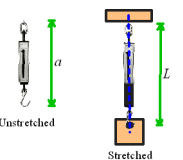The forces exerted by the ends of the spring always act along the line of the spring. The magnitude of the force is (so long as you don’t stretch the spring too much) given by the formula

F = k(L - a)

where a is the un-stretched spring length; L is the stretched length, and k is the spring stiffness.

In the SI system, k has units of N/m.

Note that when you draw a picture showing the forces exerted by a spring, you must always assume that the spring is stretched, so that the forces exerted by the spring are attractive. If you don’t do this, your sign convention will be inconsistent with the formula F = k(L - a), which assumes that a compressed spring (L<a) exerts a negative force.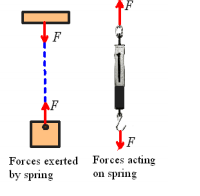Forces exerted by dashpots

A ‘Dashpot’ is somewhat like a spring except that it exerts forces that are proportional to the relative velocity of its two ends, instead of the relative displacement. The device is extremely useful for damping vibrations. The device usually consists of a plunger that forces air or fluid through a small orifice – the force required to expel the fluid is roughly proportional to the velocity of the plunger.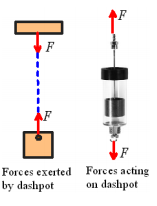The forces exerted by the ends of the dashpot always act along the line of the dashpot. The magnitude of the force exerted by a fluid filled dashpot is given by the formula

F =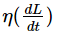where L is the length, and η is the rate constant of the dashpot. Air filled dashpots are somewhat more complicated, because the compressibility of the air makes them behave like a combination of a dashpot and spring connected end-to-end.

In the SI system, η has units of Ns/m.

Note that when you draw a picture showing the forces exerted by a dashpot, you must always assume that the length of the dashpot is increasing, so that the forces exerted by the ends of the dashpot are attractive.

Forces exerted by an ‘Inerter’

The ‘Inerter’ is a device that exerts forces proportional to the relative acceleration of its two ends. It was invented in 1997 and used in secret by the McLaren Formula 1 racing team to improve the performance of their cars, but in 2008 was made broadly available

The device is so simple that it is difficult to believe that it has taken over 100 years of vehicle design to think of it – but the secret is really in how to use the device to design suspensions than in the device itself. The device works by spinning a flywheel between two moving rods, as sketched in the figure.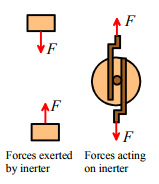The forces exerted by the ends of the inerter always act along the line of the inerter. The magnitude of the force exerted by an inerter is given by the formula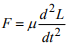where L is the length, and μ is the inertia constant of the dashpot.

In the SI system, μ has units of Ns2 /m.

Electrostatic forces

As an engineer, you will need to be able to design structures and machines that manage forces. Controlling gravity is, alas, beyond the capabilities of today’s engineers. It’s also difficult (but not impossible) to design a spring with a variable stiffness or unstretched length. But there are forces that you can easily control. Electrostatic and electromagnetic forces are among the most important ones.

Electrostatic forces are exerted on, and by, charged objects. The concepts of electrical potential, current and charge are based on experiments. A detailed discussion of these topics is beyond the scope of this course (it will be covered in detail in EN51), but electromagnetic and electrostatic forces are so important in the design of engines and machines that the main rules governing forces in these systems will be summarized here.

Electrostatic forces acting on two small charged objects that are a large distance apart

Coulomb’s Law states that if like charges Q1 and Q2 are induced on two particles that are a distance d apart, then particle 1 will experience a force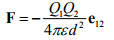(acting away from particle 2), where ε is a fundamental physical constant known as the Permittivity of the medium surrounding the particles (like the Gravitational constant, its value must be determined by experiment).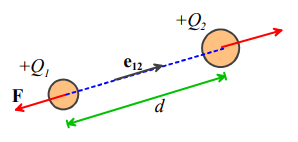In SI units, Q1,Q2, are specified in Coulombs, d is in meters, and ε is the permittivity of free space, with fundamental units Amperes2 kg-1 m-3. Permittivity is more usually specified using derived units, in Farads per meter. The Farad is the unit of capacitance.

The value of ε for air is very close to that of a vacuum. The permittivity of a vacuum is denoted by ε0 . In SI units its value is approximately  8.854185 × 10-12 Fm-1

Like gravitational forces, the electrostatic forces acting on 3D objects with a general distribution of charge must be determined using complicated calculations. It’s worth giving results for two cases that arise frequently in engineering designs:

Forces acting between charged flat parallel plates

Two parallel plates, which have equal and opposite charges Q and are separated by a distance d , experience an attractive force with magnitude

F = Q2 /(2ε)

The force can be thought of as acting at the center of gravity of the plates.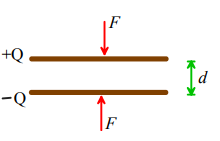Two parallel plates, which have area A, are separated by a distance d, and are connected to a powersupply that imposes an electrical potential difference V across the plates, experience an attractive force with magnitude

F =AV2ε /(2d2)

The force can be thought of as acting at the center of gravity of the plates.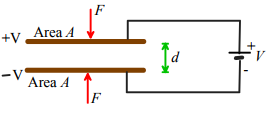Applications of electrostatic forces:

Electrostatic forces are small, and don’t have many applications in conventional mechanical systems. However, they are often used to construct tiny motors for micro-electro-mechanical systems (MEMS). The basic idea is to construct a parallel-plate capacitor, and then to apply force to the machine by connecting the plates to a power-supply. The pictures below show examples of comb drive motors.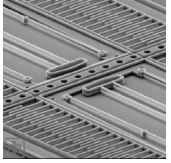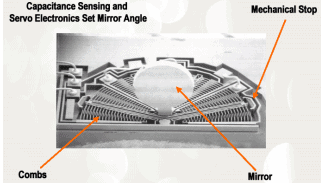An experimental comb drive MEMS actuator developed at Sandia National Labs, A rotary comb drive actuator developed. Its purpose is to rotate the mirror at the center, which acts as an optical switch.

The configurations used in practice are basically large numbers of parallel plate capacitors. A detailed discussion of forces in these systems will be deferred to future courses.

Electrostatic forces are also exploited in the design of oscilloscopes, television monitors, and electron microscopes. These systems generate charged particles (electrons), for example by heating a tungsten wire. The electrons are emitted into a strong electrostatic field, and so are subjected to a large force. The force then causes the particles to accelerate – but we can’t talk about accelerations in this course so you’ll have to take EN4 to find out what happens next…

Electromagnetic forces

Electromagnetic forces are exploited more widely than electrostatic forces, in the design of electric motors, generators, and electromagnets.

Ampere’s Law states that two long parallel wires which have length L, carry electric currents I1 and I2 , and are a small distance d apart, will experience an attractive force with magnitude

F = μ0I1 I2 L /(2πd)

where μ0 is a constant known as the permeability of free space.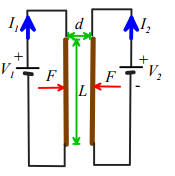In SI units, μ0 has fundamental units of  kg m2 sec-1 A -2, but is usually specified in derived units of Henry per meter. The Henry is the unit of inductance

The value of μ0 is exactly 4π ×10-7 H/m

Electromagnetic forces between more generally shaped current carrying wires and magnets are governed by a complex set of equations. A full discussion of these physical laws is beyond the scope of this course, and will be covered in EN51.

Applications of electromagnetic forces

Electromagnetic forces are widely exploited in the design of electric motors; force actuators; solenoids; and electromagnets. All these applications are based upon the principle that a current-carrying wire in a magnetic field is subject to a force. The magnetic field can either be induced by a permanent magnet (as in a DC motor); or can be induced by passing a current through a second wire (used in some DC motors, and all AC motors). The general trends of forces in electric motors follow Ampere’s law: the force exerted by the motor increases linearly with electric current in the armature; increases roughly in proportion to the length of wire used to wind the armature, and depends on the geometry of the motor.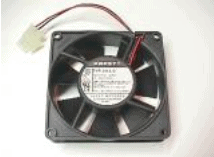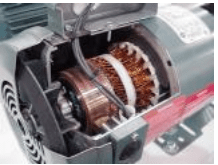Two examples of DC motors – the picture on the right is cut open to show the windings.

Offer running on EduRev: Apply code STAYHOME200 to get INR 200 off on our premium plan EduRev Infinity!

,

,

,

,

,

,

,

,

,

,

,

,

,

,

,

,

,

,

,

,

,

;